HTML 5 Physics Tools
The programs listed below are programs that I wrote for my students to use at home or in class. These programs were written to work on computers, tablets, phones and other handheld devices with HTML 5 capable browsers.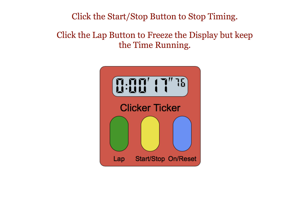Digital Stopwatch This program was created to mimic the behavior of a common type of digital stopwatch. This program can be used to demonstrate to a class how to use this type of stopwatch or it can be used as a stopwatch to time live events. YouTube video describing the tool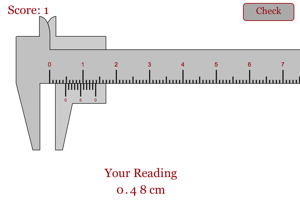Reading a Caliper This program was designed to make sure students can read a Vernier Caliper. Students will be presented with different caliper setups and they must be able to correctly answer four in a row in order to start working on the lab using calipers.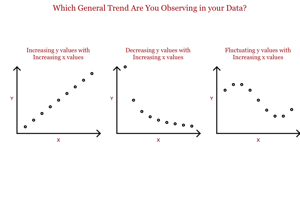Help With Graph Types This program was designed to make sure students can properly curve fit the different types of data they will run across in class. YouTube video describing the tool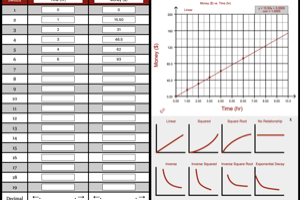Quick Graph This program was designed as a quick graphing program that students could use on a computer, tablet or smart phone when a more complex graphing program is not available. This program will let students plot up to 10 data points and then get a best fit to those points. It works with linear, inverse, inverse square, square, square root, no relationship and exponential decay graphs. YouTube video describing the tool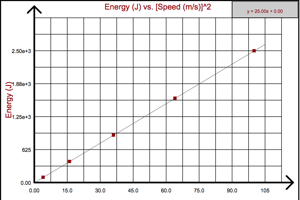Quick Graph and Linearization This program was designed as a quick graphing program that students could use on a computer, tablet or smart phone when a more complex graphing program is not available. This program will let students plot up to 10 data points and then transform the columns of data to linearize the graph. YouTube video describing the tool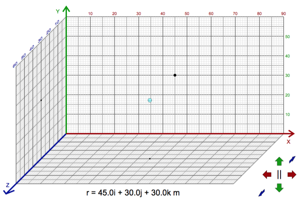3D Position Vectors This program's sole purpose is help students visualize position vectors in three dimensions. Students will be able to move a ball around in a room and they will be shown the position vector for the location of the ball relative to the bottom corner of the room. YouTube video describing the tool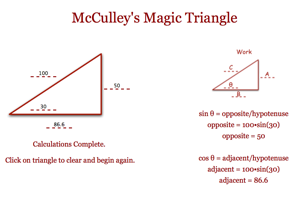Magic Triangle This program will allow students to quickly break a vector into components or turn the vector components into resultant vector. YouTube video describing the lab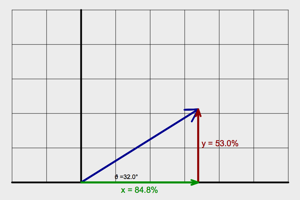Trig Stuff This program gives students a visual representation of sine and cosine at different angles. A unit vector will cycle through different angles and students can see the changes that take place to the horizontal part and the vertical part of the vector. They will be introduced to sine as the percent of the hypotenuse for the opposite leg of the triangle and cosine as the percent of the hypotenuse for the adjacent leg. They will see that the percentages add to 100 percent when added in a pythagorean sense. YouTube video describing the lab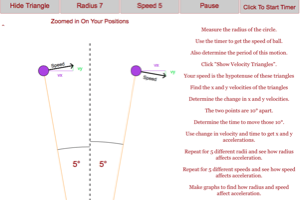Circular Acceleration This program gives students a visual representation of why an object moving in a circle at constant speed is accelerating. This program will guide students through a method of calculating the circular acceleration based on the change in velocity at two nearby positions. Students will be able to change radius and speed to see how each factor affects the circular acceleration. YouTube video describing the lab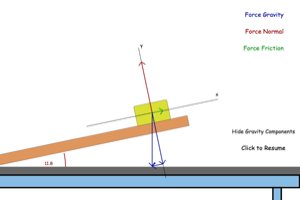Visualizing Forces on an Incline This program gives students a visual representation of the forces on a block that is on an inclined plane. The students can watch how force gravity, force normal, and force friction change as the angle of the plane changes. Students can also look at the components of force gravity. YouTube video describing the lab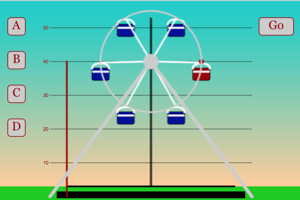Visualizing Sine Curves This program gives students a visual representation of sine curves. The hope is that students can relate properties of the ferris wheel to properties of the sine curve. The height of the center of the ferris wheel is their vertical shift, the speed of the ferris wheel determines the B value, the radius of the ferris wheel translates to the amplitude of the curve and the C, or phase shift, is determined by the starting location of the ferris wheel. YouTube video describing the labVisualizing A Wave as Multiple Oscillating Masses This program gives students a visual representation of a wave as a line of masses that are oscillating out of step. Each oscillating mass will affect the oscillation of the wave next to it. YouTube video describing the lab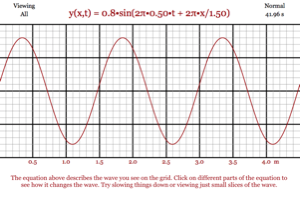Visualizing the Wave Equation This program gives students a visual representation of the wave equation. This will let students see how all the different variables come together to create a wave that propagates through space and time. YouTube video describing the lab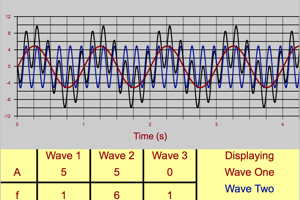Combining Waves This program gives students a way to combine waves together to see what properties the new wave will possess. It is helpful in looking at constructive and destructive interference. It can also illustrate beats and carrier waves. YouTube video describing the lab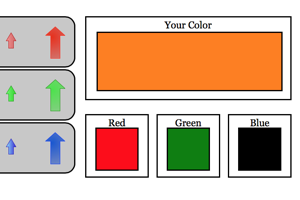Color Mixing Activity This program gives students a chance to get a feel for the different colors that can be made by mixing red, green and blue light in different proportions. This activity is meant to be a lead in to students using the color mixing game program. YouTube video describing the lab